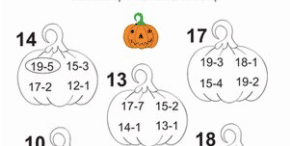# Use Pumpkins Not Clipart

Holiday worksheets do not make learning more fun. It’s marginally better than just a page of those math problems without the pumpkins, but why not give students more authentic learning opportunities? Instead of clip art pumpkins let’s have kids use real pumpkins! Dave Burgess says creativity is not an inherent trait, it is something you practice. So practice some creative ideas for using pumpkins in a lesson. Below is a crowdsourced spreadsheet you can add your idea to!

## Pumpkin Ideas### 4 C’s

Which of the 4 C’s does this worksheet have? Creativity, no. Critical thinking, no. Clearly communicating ideas, no. Collaboration, no. If students are doing low critical thinking questions they should at least get immediate feedback (make this digital) and the teacher can collect good data to better support struggling students or see which students need more challenge. What improves learning is not the teacher spending time marking these pumpkin math problems right and wrong. High quality feedback that is actionable improves learning. Provide students more challenging questions or problems

Clipart is NOT one of the 4 C’s

### Use Real Data

When designing lessons we should ask “why do the kids care about this?” The response of “because it is important” is not an answer. This is the art of teaching, designing lesson plans for the students you have that engage them to make learning relevant and sticky. Will students remember filling out this pumpkin worksheet years from now? Probably not, but they will remember using real pumpkins!

### Higher Critical Thinking

The website openmiddle.com provides examples of DOK 2 and DOK 3 level math problems. Here is one by Robert Kaplinsky on subtraction that is DOK 3 (strategic thinking) as opposed to the DOK 1 level math problems in the pumpkin worksheet.

### 8 Mathematical Principles

Not only does this pumpkin worksheet not have the any of the 4 C’s or an authentic context it does NOT address any of the 8 mathematical principles.  A math lesson should have the students doing at least one of the 8 practices.

#### Common Core

Notice the standard is to use strategies. Ask students to communicate their ideas and thinking.

CCSS 1.OA.6 – Add and subtract within 20, demonstrating fluency for addition and subtraction within 10. Use strategies such as counting on; making ten (e.g., 8 + 6 = 8 + 2 + 4 = 10 + 4 = 14); decomposing a number leading to a ten (e.g., 13 – 4 = 13 – 3 – 1 = 10 – 1 = 9); using the relationship between addition and subtraction (e.g., knowing that 8 + 4 = 12, one knows 12 – 8 = 4); and creating equivalent but easier or known sums (e.g., adding 6 + 7 by creating the known equivalent 6 + 6 + 1 = 12 + 1 = 13).

CCSS 2.NBT.5 – Fluently add and subtract within 100 using strategies based on place value, properties of operations, and/or the relationship between addition and subtraction.

CCSS 2.NBT.7 – Add and subtract within 1000, using concrete models or drawings and strategies based on place value, properties of operations, and/or the relationship between addition and subtraction; relate the strategy to a written method. Understand that in adding or subtracting three-digit numbers, one adds or subtracts hundreds and hundreds, tens and tens, ones and ones; and sometimes it is necessary to compose or decompose tens or hundreds.

## Subscribe to the Alice Keeler Newsletter